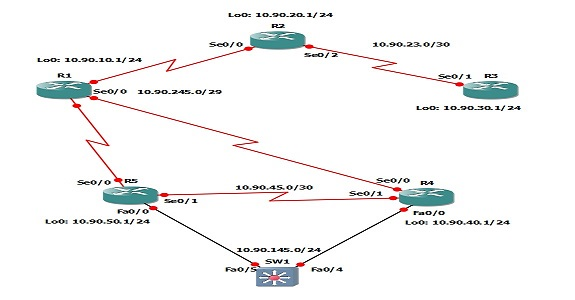## What is OSPF Metric Value Cost, Default Cost Reference Bandwidth

The term cost used by the OSPF(Open Shortest Path First) refers to the value of the matrix. Also, another feature called Reference Bandwidth is also used by the OSPF that is mainly used for the purpose of cost calculation with 100Mbps. To know more about What is OSPF Metric Value Cost, Default Cost Reference Bandwidth, keep reading this article till the end.

The cost can be calculated using the following equation:

Reference bandwidth / Interface Bandwidth.

To understand this, we can go through an example. Consider the reference bandwidth is 20Mbps Ethernet. Then by using the above equation, the cost can be calculated as:

100Mbps/20Mbps=5.

Here, we take the value of reference bandwidth as 100Mbps. Because its default value is 100Mbps. There will be a less overhead while the packets are passed through this link than we transmitting the same packets through 56kbps serial link. The cost value is calculated by using this logic. The reference bandwidth can be set or can be changed as our wish and this value is indicated in Mbps. If we want to see the reference bandwidth in cisco routers, then we can use the following command for that purpose:

R1 # show IP OSPF | i      band.

If the bandwidth is high, then the cost value will be less. Similarly, if the interface bandwidth is too small, then the corresponding cost value will be greater. Also, based on this calculation of OSPF cost, there is a default value for the cost of the Gigabit Ethernet interface. We can say that the default value for the Fast Ethernet Interface’s OSPF cost is, 100Mbps and the default value for the Gigabit Ethernet Interface’s OSPF cost value is 1Mbps.

If someone wants to change this default value, it can be achieved by adjusting the cost formula. It can be adjusted under the OSPF routing process by using the command auto-cost. We should care that, when we changing the default OSPF reference bandwidth, one thing must follow, that is, this updation must be reflected in the OSPF reference bandwidth of all OSPF  Otherwise, it will lead some errors.

We can make a table containing the Bandwidth and their corresponding Cost value:

 Interface Bandwidth OSPF default Cost value 100 Gbps 1 40 Gbps 1 10 Gbps 1 1 Gbps 1 100 Mbps 1 10 Mbps 10 1.544 Mbps 64 768 Kbps 133 384 Kbps 266 128 Kbps 781

If the cost value is a decimal value, then we can round it only to backward, because there is a rule that the cost value should always be a positive integer. And also if the cost value is less than the value of 1, it will also be treated as the value 1.